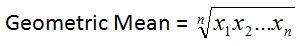# Geometric Mean: Definition and Formula

An error occurred trying to load this video.

Try refreshing the page, or contact customer support.

Coming up next: Angle Bisector Theorem: Definition and Example

### You're on a roll. Keep up the good work!

Replay
Your next lesson will play in 10 seconds
• 0:01 Geometric Mean
• 0:37 Uses
• 1:38 Formula
• 2:29 Using the Formula
• 4:28 Lesson Summary
Save Save

Want to watch this again later?

Timeline
Autoplay
Autoplay
Speed Speed

#### Recommended Lessons and Courses for You

Lesson Transcript
Instructor: Yuanxin (Amy) Yang Alcocer

Amy has a master's degree in secondary education and has taught math at a public charter high school.

Watch this video lesson to learn how different the geometric mean is from the arithmetic mean, or average, that we are all so familiar with. Learn in what circumstance the geometric mean is preferred. Also, learn the formula and how to use it.

## Geometric Mean

The geometric mean is the nth root when you multiply n numbers together. It is not the same as the arithmetic mean, or average, that we know. For the arithmetic mean, we add our numbers together and divide by how many numbers we have. The geometric mean uses multiplication and roots. For example, for the product of two numbers, we would take the square root. For the product of three numbers, we take the third root.

## Uses

When would we use the geometric mean as opposed to the arithmetic mean? We would use the geometric mean when we want to figure out the average rate of growth if the growth rate is determined by multiplication. For example, the average percentage amount of growth in a bank account per year uses the geometric mean since the growth each year is determined by multiplying the amount in the bank account by the percentage growth.

The arithmetic mean is used when the growth is determined by addition. For example, the average amount it costs to feed people at a party uses the arithmetic mean because the growth in cost is determined by addition. The geometric mean does have one limitation - it cannot be used for negative numbers; all the numbers have to be positive. You can, however, find the geometric mean for as many numbers as you want.

## Formula

The formula to find the geometric mean is this:It tells us to first multiply all the numbers we have together and then to take the nth root of that product. If we had two numbers, we take the square root; if we have four numbers, we take the fourth root. Most calculators have a button next to the square root button that lets you choose the root. This button lets you calculate the third root, fourth root, fifth root, etc. - whichever root you need. Now some calculators will have you choose the root before pushing the button, while others have you choose the root after pushing the button. Get familiar with your calculator first so you know how yours will calculate your roots.

## Using the Formula

Let's look at some examples to see how we can use this formula. Say we want to find the geometric mean of 3, 4, and 9. What does the formula tells us to do first? It tells us to multiply our numbers together first. So let's do that: 3 * 4 * 9 = 108. Then the formula tells us to take the third root since we have three numbers. Doing that, we get 4.7622 for our answer.

To unlock this lesson you must be a Study.com Member.

### Register to view this lesson

Are you a student or a teacher?

#### See for yourself why 30 million people use Study.com

##### Become a Study.com member and start learning now.
Back
What teachers are saying about Study.com

### Earning College Credit

Did you know… We have over 200 college courses that prepare you to earn credit by exam that is accepted by over 1,500 colleges and universities. You can test out of the first two years of college and save thousands off your degree. Anyone can earn credit-by-exam regardless of age or education level.